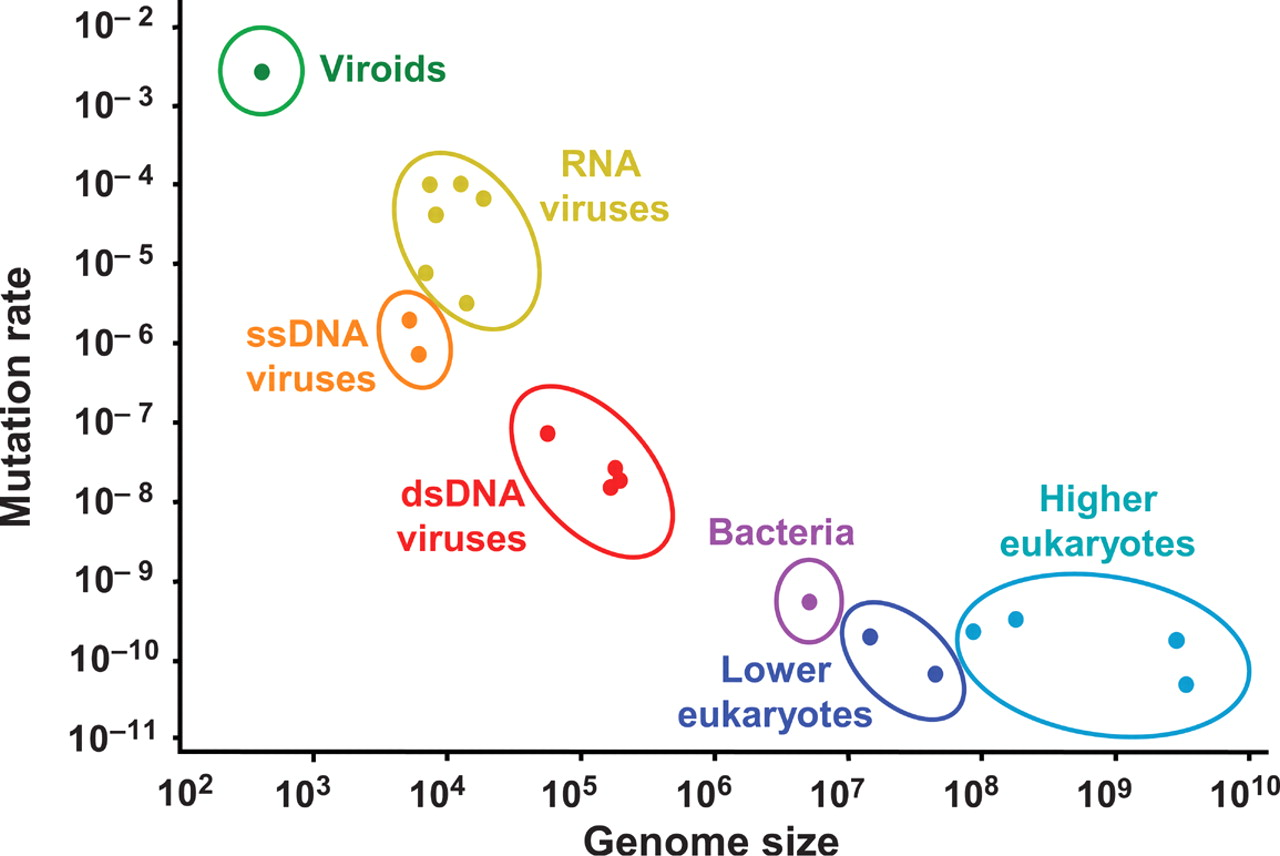Richard Neher
Biozentrum, University of Basel

## Why be accurate?

• Much of biology relies on error free synthesis of very long polymers (DNA, proteins)
• If the per base/aa error rate is $\epsilon$, probability of an error free product is $e^{-\epsilon L}$
• Error free replication of a bacterial chromosome ($10^7$ bases) requires error rates $\ll 10^{-7}$

### What limits accuracy?

#### Michaelis-Menten reaction

$S + E \leftrightharpoons ES \rightarrow P + E$
• Biochemical reactions typically go through intermediate states.
• On rates are similar, limited by diffusion.
• Discrimination is via the off-rates
• Off-rates determine the life time of the intermediate.
• Off-rates are determined by the free energy of the transition state
• The ratio of off-rates is given by $e^{-\Delta G/kT}$. Lower limit for error rate!
• Typical energies of discrimination are low.
• DNA base pairing/stacking energies $\sim 3kT$
• Translation is based on mRNA/anti-codon interaction. Mismatches cost a few $kT$.John Hopfield
Princeton UniversityJaques Ninio
ENS Paris

### Error rates of genome replication### Self/non-self antigen discrimination by T-cells

• T-cells scan MHC/peptide complexes for evidence infection.
• Many self-antigens, few non-self.
• Interactions energies are similar.
• Need for rapid but accurate discrimination.T cell receptor binding to MHC-antigen complex. Courtesy of J. Kimball